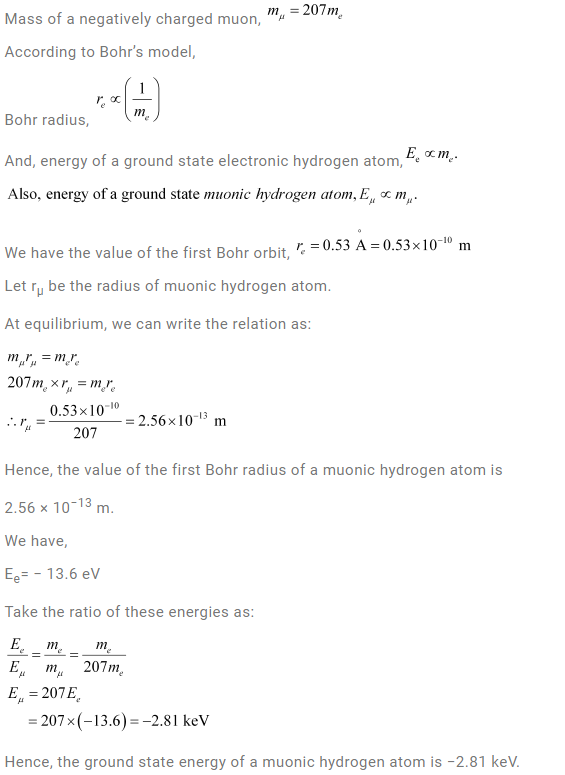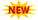SSC Phase 10 Notification || TSSPDCL AE Notification || PPSC Naib Tehsildar Admit Card ||

# NCERT Solutions For Class 12 Physics Chapter 12 Atoms

NCERT Solutions For Class 12 Physics Chapter 12 discusses the problems with Rutherford’s Alpha particle scattering experiment, Atomic Spectra such as Balmer Series, Bohr’s Atomic Model, etc. Atoms Class 12 NCERT Solutions gives students an in-depth understanding of the inner workings of the atomic model, De-Broglie’s Explanation of Bohr’s Second Postulate of Quantization, JJ Thompson’s Atomic model, and its limitations. Students can find solved problems in other chapters on the page NCERT Solutions For Class 12 Physics. For Other NCERT Solutions, we suggest students visit the NCERT Solutions page. This Class 12 Physics Chapter 12 Solutions covers topics such as Line Spectra like Lyman Series, Paschen Series, Brackett Series, and Pfund series are introduced. Why atoms are considered to be composed mostly of empty space is also answered here.

## Class 12 NCERT Physics Chapter 12 Solutions

Subtopics in the NCERT Solutions For Class 12 Physics Chapter 12 are given below:

 Section Topic Name 12 Atoms 12.1 Introduction 12.2 Alpha-particle Scattering and Rutherford’s Nuclear Model of Atom 12.3 Atomic Spectra 12.4 Bohr Model of Hydrogen Atom 12.5 The line spectra of Hydrogen Atom 12.6 De Broglie’s Explanation of Bohr’s Second Postulate of Quantization

### Class 12 Physics Atoms Chapter 12 –  NCERT Solutions

Question 12.1: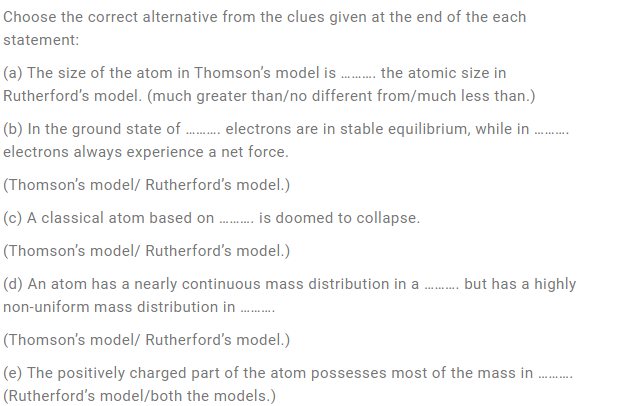Solution: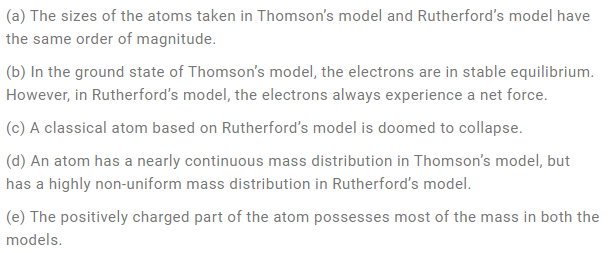Question 12.2:Solution: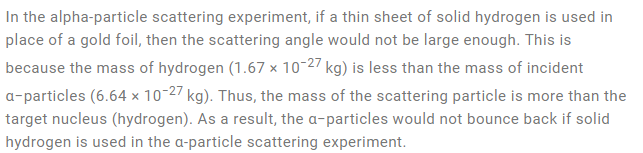Question 12.3: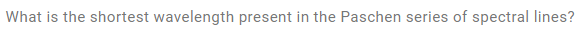Solution: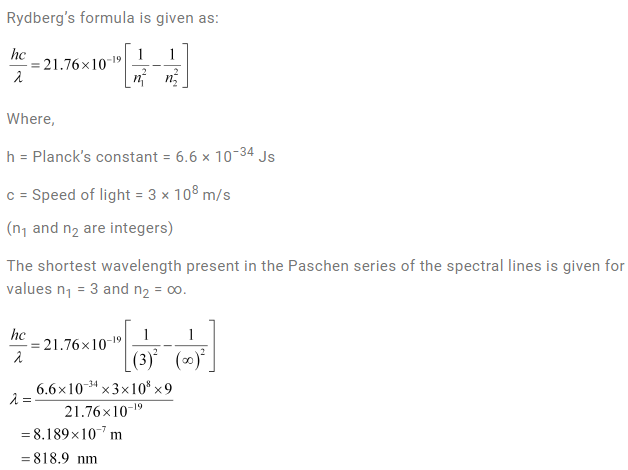Question 12.4: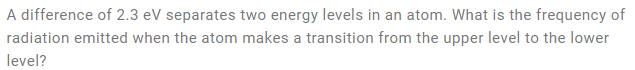Solution: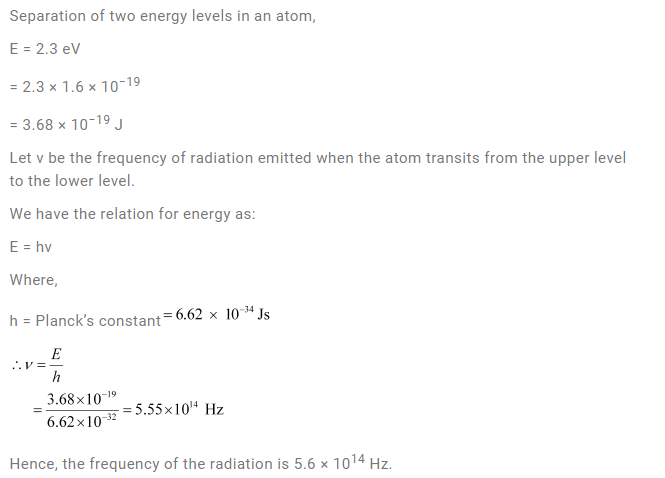Question 12.5: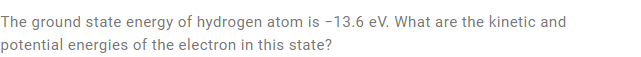Solution: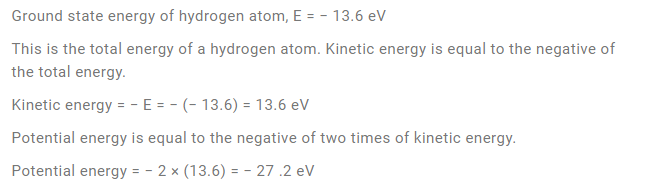Question 12.6: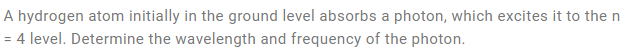Solution: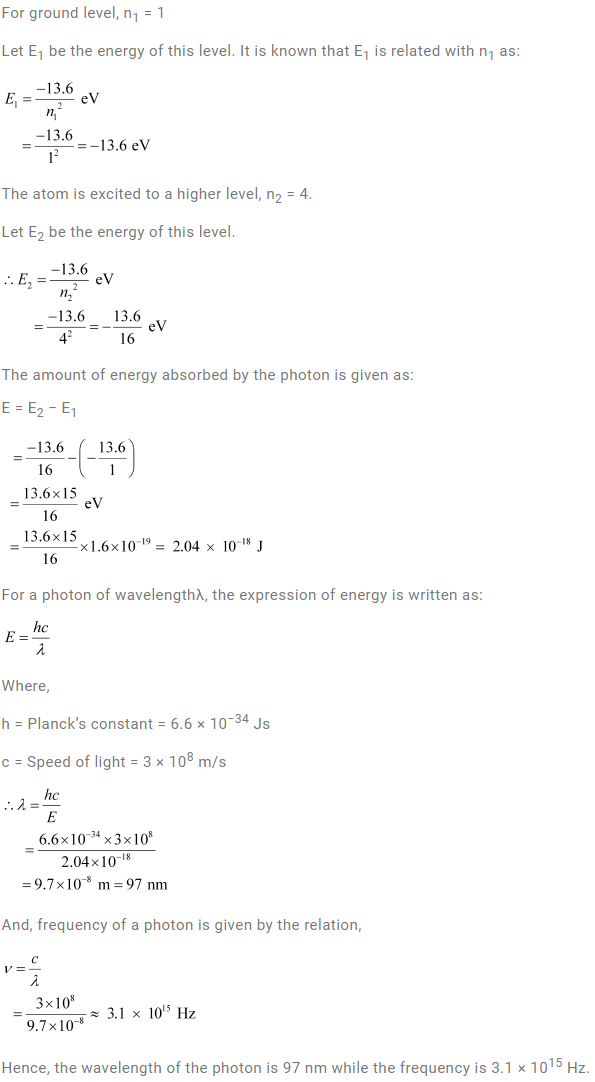Question 12.7: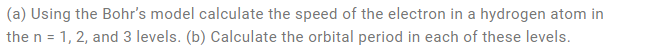Solution: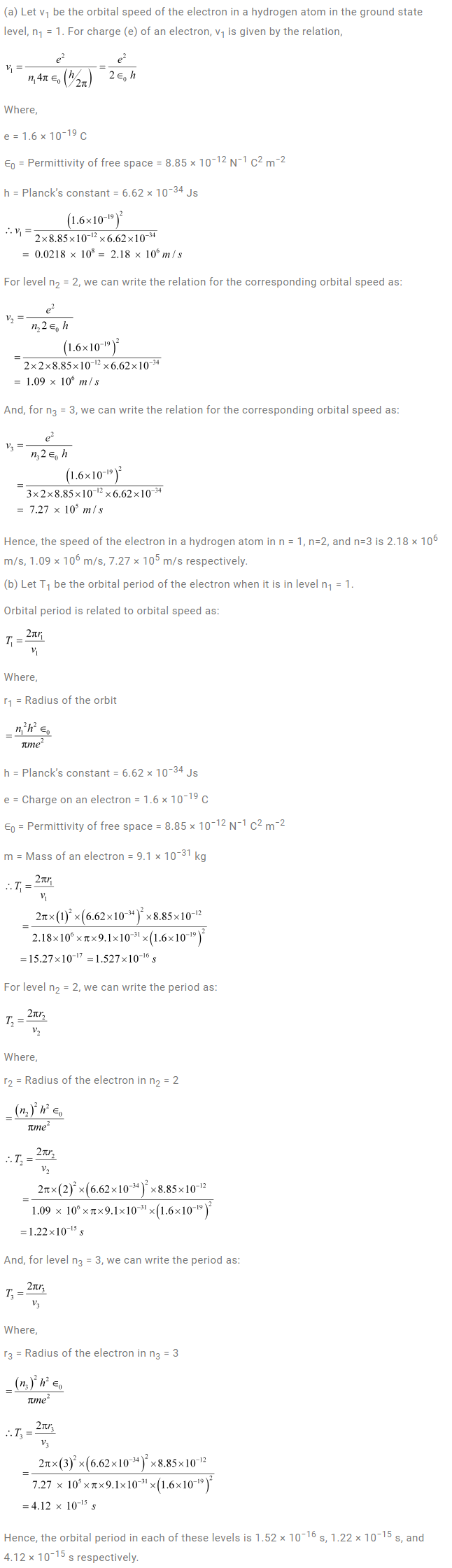Question 12.8: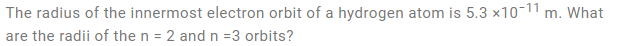Solution: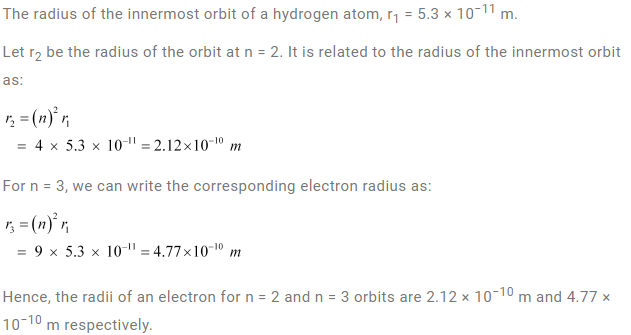Question 12.9: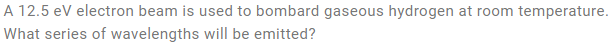Solution: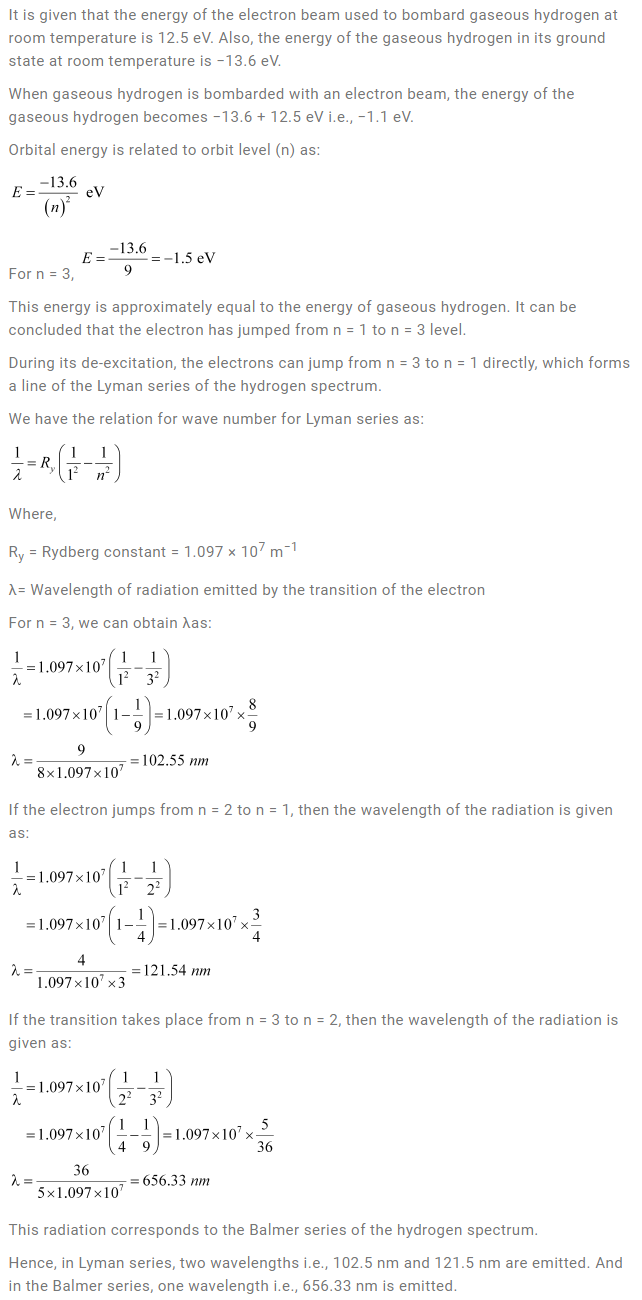Question 12.10: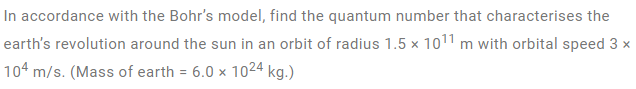Solution: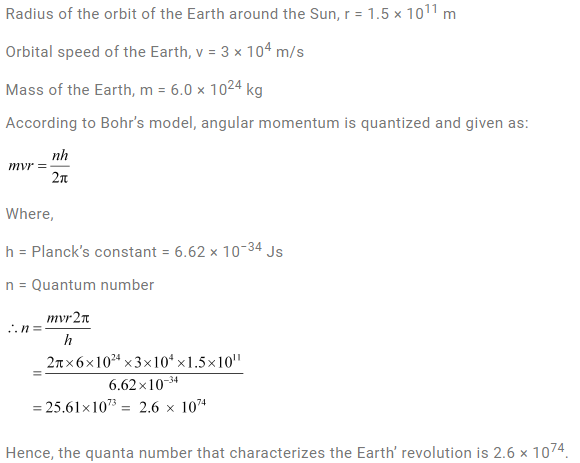Question 12.11: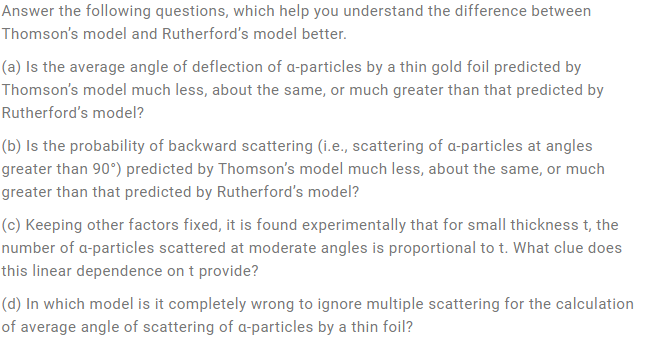Solution: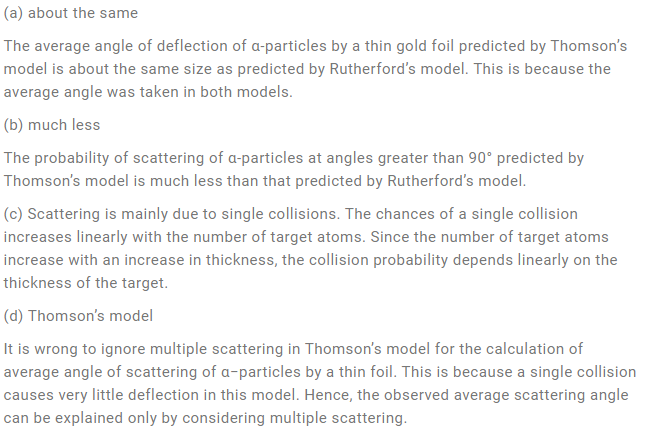Question 12.12: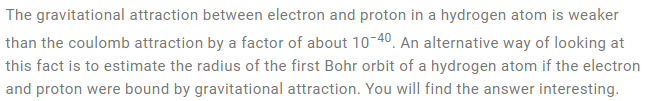Solution: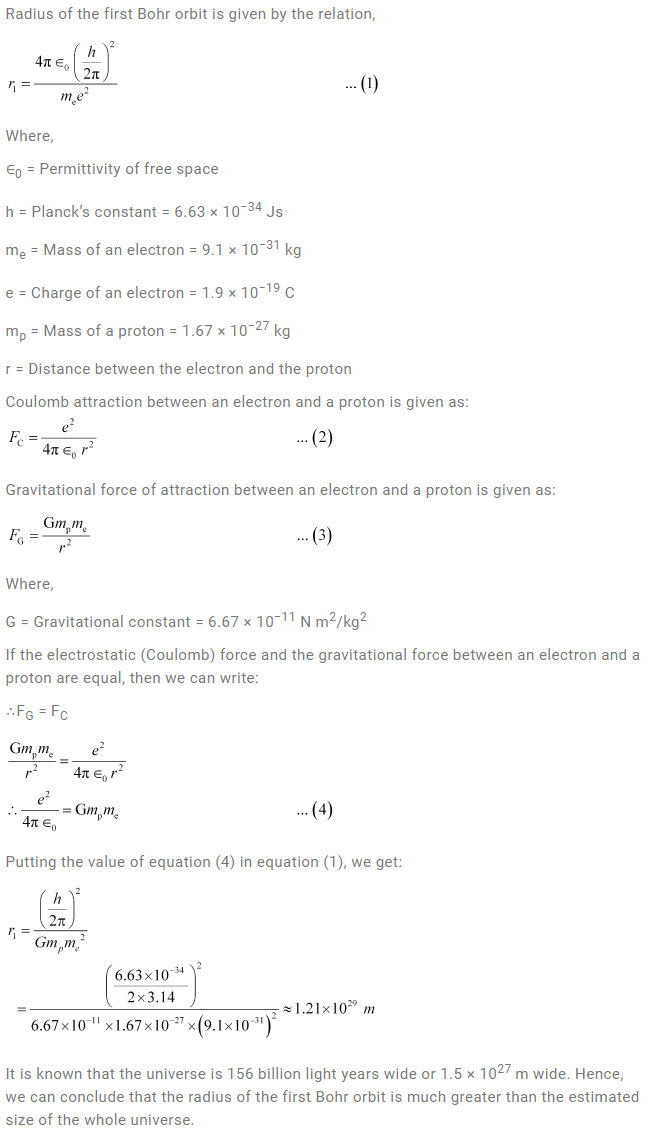Question 12.13:Solution: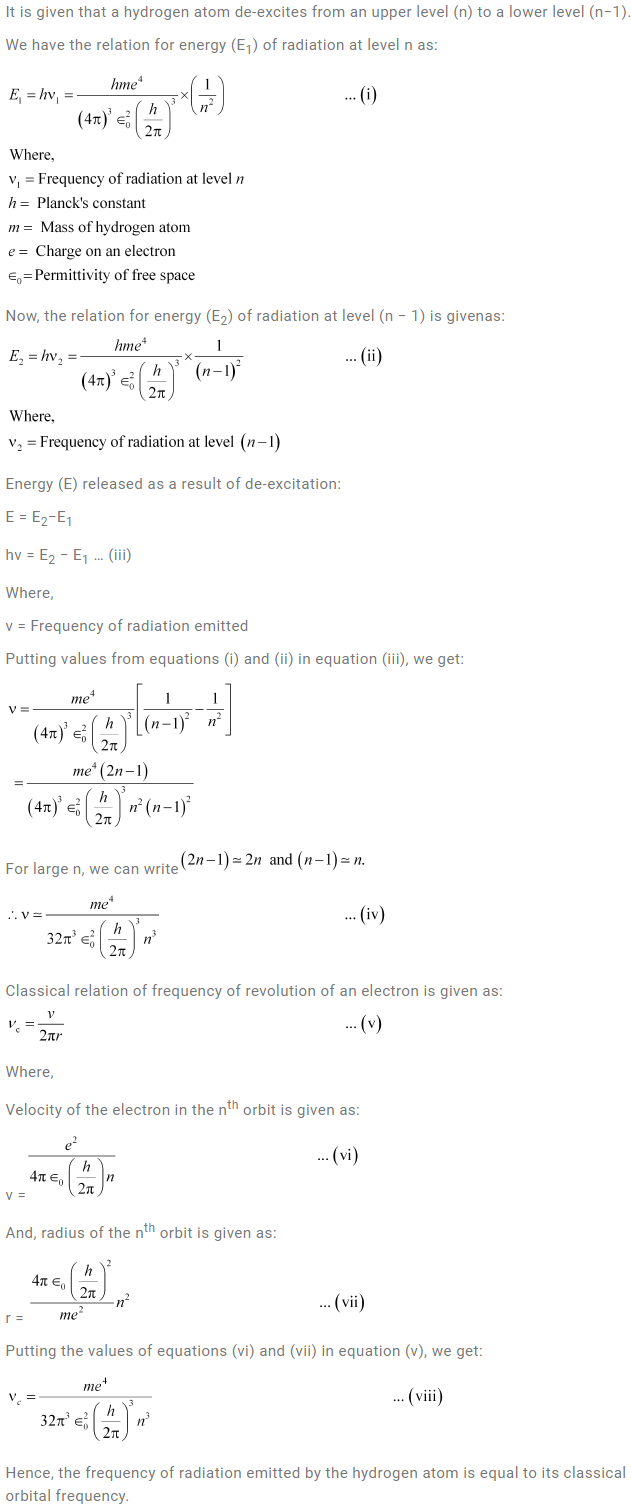Question 12.14: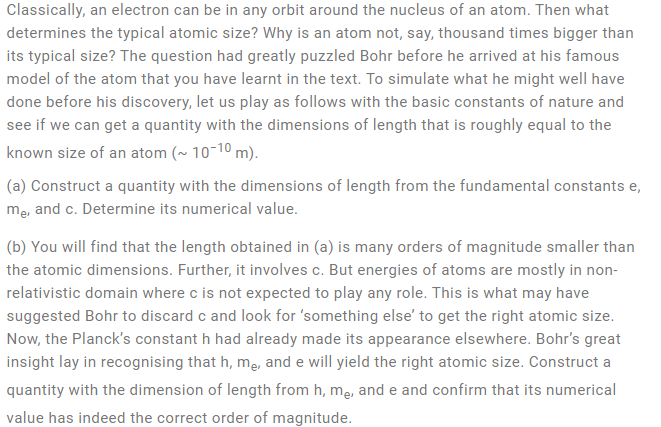Solution: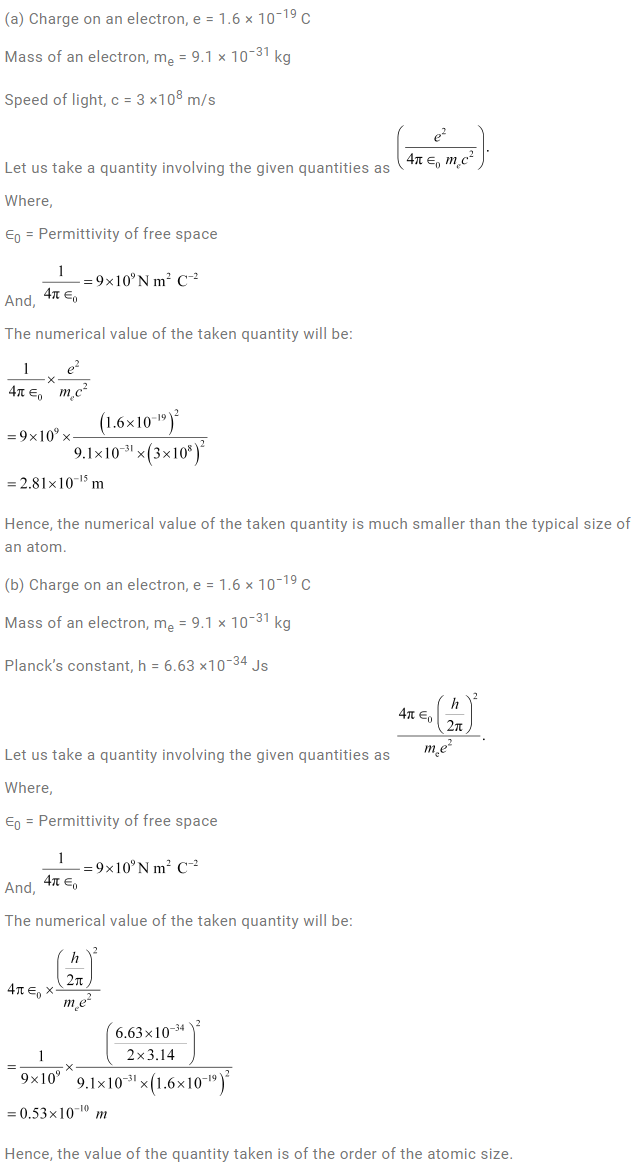Question 12.15: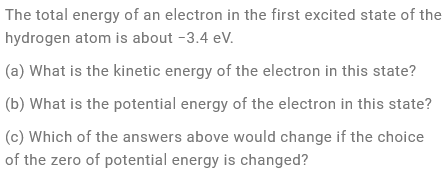Solution: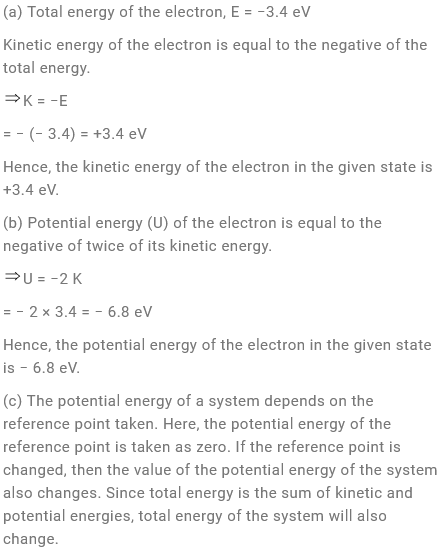Question 12.16: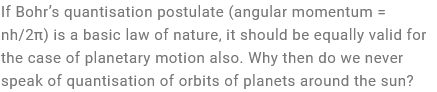Solution: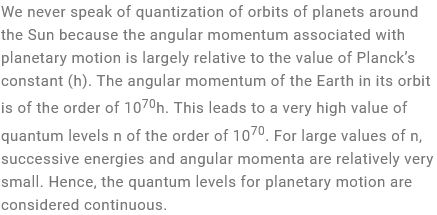Question 12.17: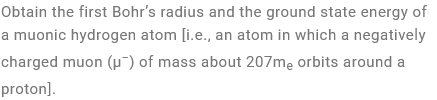Solution: